Here you will find summaries, formula, explanation and more on O Level Additional Mathematics.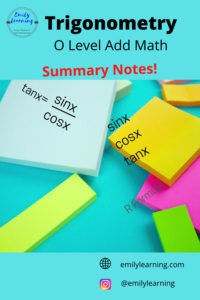## Summary Notes for Trigonometry – O Level Add Math

Here’s a summary of Trigonometry for O Level Additional Mathematics. I go through what’s tested in this  article here.  Assumed Trigonometry Knowledge for O Level Add…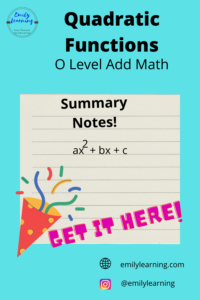Here, you’ll find a summary of what’s tested in the Quadratic function for O Level Additional Mathematics. If you are interested to know what’s in the…## Summary Notes for Binomial Expansion – O Level Add Math

In this post, I’ll share with you a summary of binomial expansion based on the O Level Additional Mathematics syllabus. You can find what’s covered…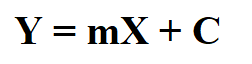## Summary Notes for Linear Law – O Level Add Math

Linear law is a chapter that revolves around making a non-linear relationship linear by changing our concept of y must be the vertical axis and…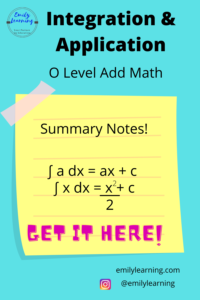## Summary Notes for Integration and its Application – O Level Add Math

Here, you’ll find the summary notes for integration and application of integration written based on what’s tested in the O Level Add Maths syllabus. In…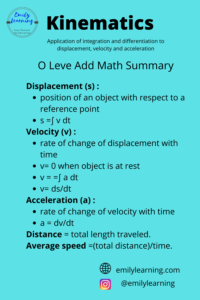## Summary Notes for Kinematics – O Level Add Math

Here, you’ll find the summary notes for kinematics written based on the O Level Add Maths syllabus. In my previous articles, I talked in detail here about…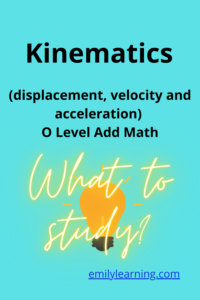## What you need to know for kinematics (displacement, velocity and acceleration) as tested in O Level Add Math

Application of integration and differentiation to kinematics is a chapter most schools cover after they completed all the chapters on integration and differentiation. Usually, one…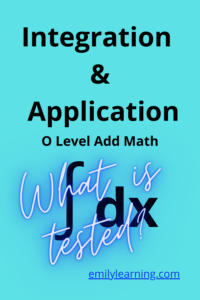## What you need to know for integration and its application as tested in O Level Additional Mathematics

Integration is a big topic in O Level Additional Mathematics, just like differentiation. Integration and differentiation make up what often call calculus. Just like differentiation, you are…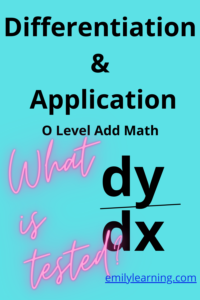## What you need to know for Differentiation and its application tested in O Level Additional Mathematics

Differentiation is a big topic in O Level Additional Mathematics. If you are using the textbook, you will notice the many chapters (and pages) devoted…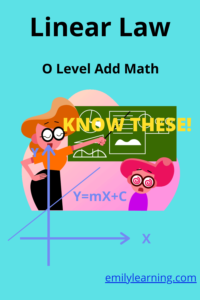## What is tested for linear law tested in O Level Additional Mathematics

Let’s talk about what you need to know about linear law in O Level Additional Mathematics. Basically, in this topic, you are required to convert…

error: Content is protected !!